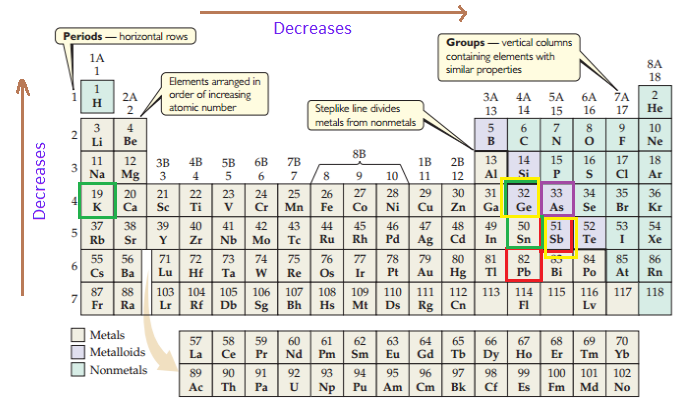×
Get Full Access to Introductory Chemistry - 5 Edition - Chapter 9 - Problem 86p
Get Full Access to Introductory Chemistry - 5 Edition - Chapter 9 - Problem 86p

×

# Choose the more metallic element from each pair.(a) Sb or Pb(b) K or Ge(c) Ge or Sb(d)ISBN: 9780321910295 34

## Solution for problem 86P Chapter 9

Introductory Chemistry | 5th Edition

• Textbook Solutions
• 2901 Step-by-step solutions solved by professors and subject experts
• Get 24/7 help from StudySoup virtual teaching assistantsIntroductory Chemistry | 5th Edition

4 5 1 431 Reviews
29
4
Problem 86P

Choose the more metallic element from each pair.

(a) Sb or Pb

(b) K or Ge

(c) Ge or Sb

(d) As or Sn

Step-by-Step Solution:

Step 1 of 5

Here, we have to choose the more metallic element from each pair. This is explained by the periodic table. In periodic table, metallic character decreases as we move across the table from left to right, and decreases as we move from bottom to top. Based on this let’s identify the more metallic element in each pair.Step 2 of 5

Step 3 of 5

##### ISBN: 9780321910295

Introductory Chemistry was written by and is associated to the ISBN: 9780321910295. Since the solution to 86P from 9 chapter was answered, more than 5830 students have viewed the full step-by-step answer. The full step-by-step solution to problem: 86P from chapter: 9 was answered by , our top Chemistry solution expert on 05/06/17, 06:45PM. This textbook survival guide was created for the textbook: Introductory Chemistry, edition: 5. The answer to “?Choose the more metallic element from each pair.(a) Sb or Pb(b) K or Ge(c) Ge or Sb(d) As or Sn” is broken down into a number of easy to follow steps, and 20 words. This full solution covers the following key subjects: choose, element, metallic, pair. This expansive textbook survival guide covers 19 chapters, and 2046 solutions.

## Discover and learn what students are asking

Calculus: Early Transcendental Functions : Product and Quotient Rules and Higher-Order Derivatives
?Using the Product Rule In Exercises 1–6, use the Product Rule to find the derivative of the function. $$g(x)=\left(x^{2}+3\right)\left(x^{2}-4 x\ Calculus: Early Transcendental Functions : The Natural Logarithmic Function: Integration ?In Exercises 1-26, find the indefinite integral. \(\int \frac{4 x^{3}+3}{x^{4}+3 x} d x$$

Statistics: Informed Decisions Using Data : Comparing Three or More Means (One-Way Analysis of Variance)
?Which Delivery Method Is Best? At a community college, the mathematics department has been experimenting with four different delivery mechanisms for c

Unlock Textbook Solution